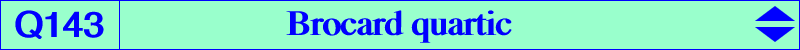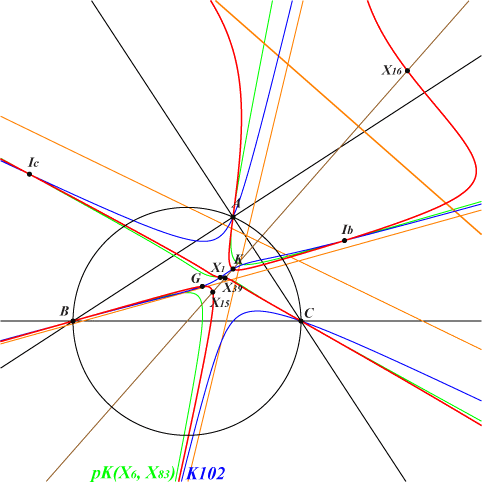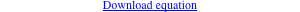∑ a^4 (b^2 + c^2) (c^2 y^2 - b^2 z^2) y z = 0A, B, C which are inflexion points X(1), X(2), X(6), X(15), X(16), X(39), X(512) excenters 6 feet of bisectors infinite points of the Grebe cubic K102Q143 is the locus of P such that X(39), P and P*/P are collinear. See Q002, locus property 9, for other similar curves. The 3rd polar curve of X(39) in Q143 is pK(X6, X83) hence the tangents to Q143 at A, B, C and the in/excenters concur at X(39). The fourth point Po of Q143 on the line X(1)X(39) is simple with SEARCH = 151.949838639611. Po = a(b+c)(a^2+bc)-2a^2(b^2+c^2) : : . Its three extraversions also lie on Q143 and on the lines passing through X(39) and an excenter.